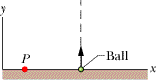# Angular Momentum of Shot Question

• Ghostscythe
In summary, the problem involves a ball being shot upward with an initial speed of 37.5 m/s and a mass of 0.360 kg. The question asks for the angular momentum at two specific points: at maximum height and halfway back to the ground at a distance of 2.15 m horizontally from the launch point. Using the equations l = r_{\bot}mv and v^2 = v^{2}_{0} + 2a(x - x_{0}), the angular momentum was found to be 0 at maximum height and 20.52 N*m*s at the halfway point.

## Homework Statement

In the figure below, a 0.360 kg ball is shot directly upward at initial speed 37.5 m/s.What is its angular momentum about P, 2.15 m horizontally from the launch point, when the ball is at the following heights?
• a) its maximum height
• b) halfway back to the ground

## Homework Equations

$\stackrel{\rightarrow}{l} = m(\stackrel{\rightarrow}{r} \times \stackrel{\rightarrow}{v})$, which given this problem simplifies to $l = r_{\bot}mv$
$v^2 = v^{2}_{0} + 2a(x - x_{0})$

## The Attempt at a Solution

For a), v was obviously 0 at max height, so the angular momentum was 0 as well.

For b):
First, I needed to find the halfway position in regards to y, to help find the velocity there. Taking:
* $v_{initial}$ = the time just after being launched from the ground
* $v_{final}$ = the apex of its climb

I plugged in the numbers: $0^2 = 37.5^2 + 2(-9.8)(y - 0)$ and got: $y = 71.747$

Now using the same equation, except with $v_{final}$ being halfway up and y obviously half of what it was:
$v^2 = 0^2 + 2(9.8)(35.874 - 0)$

I got $v^2 = 703.125$ so v = -26.517 m/s.

Edit: Plug in the numbers, and $(-26.517 m/s)(2.15 m)(0.36 kg) = 20.52$ N*m*s, which is right.

Not sure what I did the first time, but thanks, I guess having to do the post format for this forum helps, haha!

Last edited: